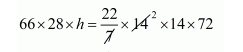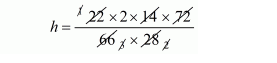# A cylindrical bucket 28 cm in diameter and 72 cmQuestion:

A cylindrical bucket 28 cm in diameter and 72 cm high is full of water. The water is emptied into a rectangular tank 66 cm long and 28 cm wide. Find the height of the water level in the tank.

Solution:

Let h be the height of rectangular tank = volume of cylindrical bucket

$66 \times 28 \times h=\pi\left(\frac{28}{2}\right)^{2} \times 72$$h=24 \mathrm{~cm}$

Hence, the height of rectangular tank is 24 cm.